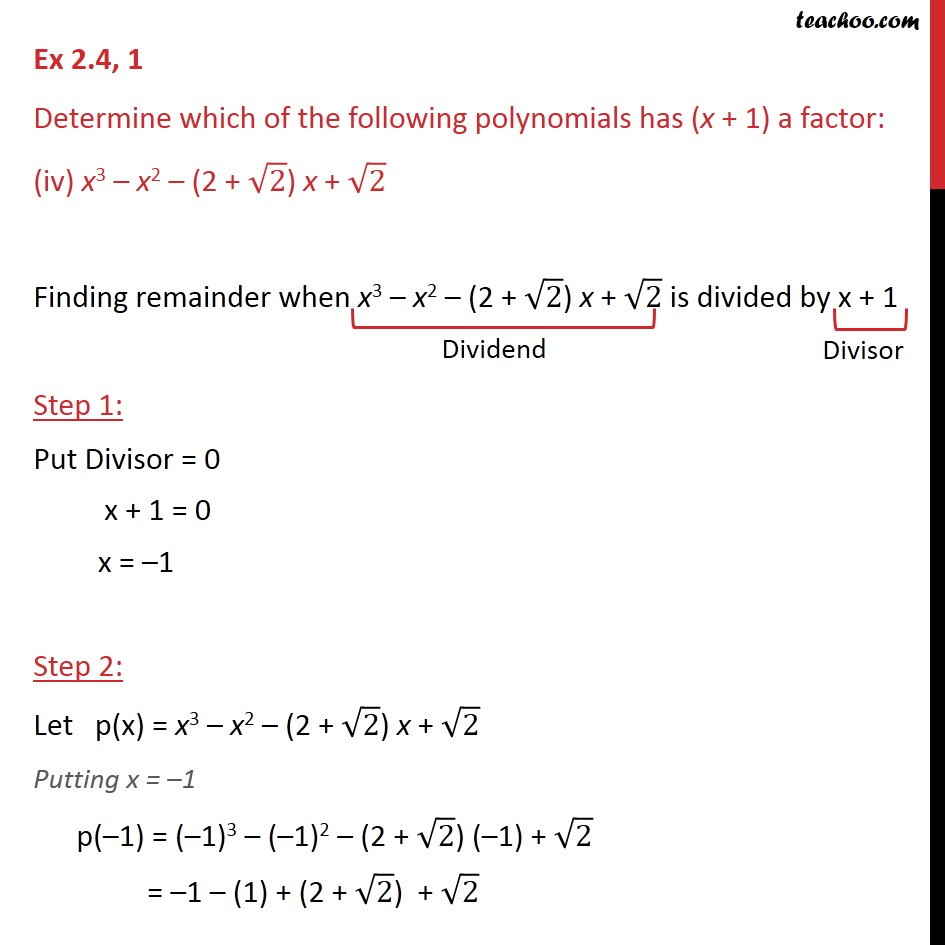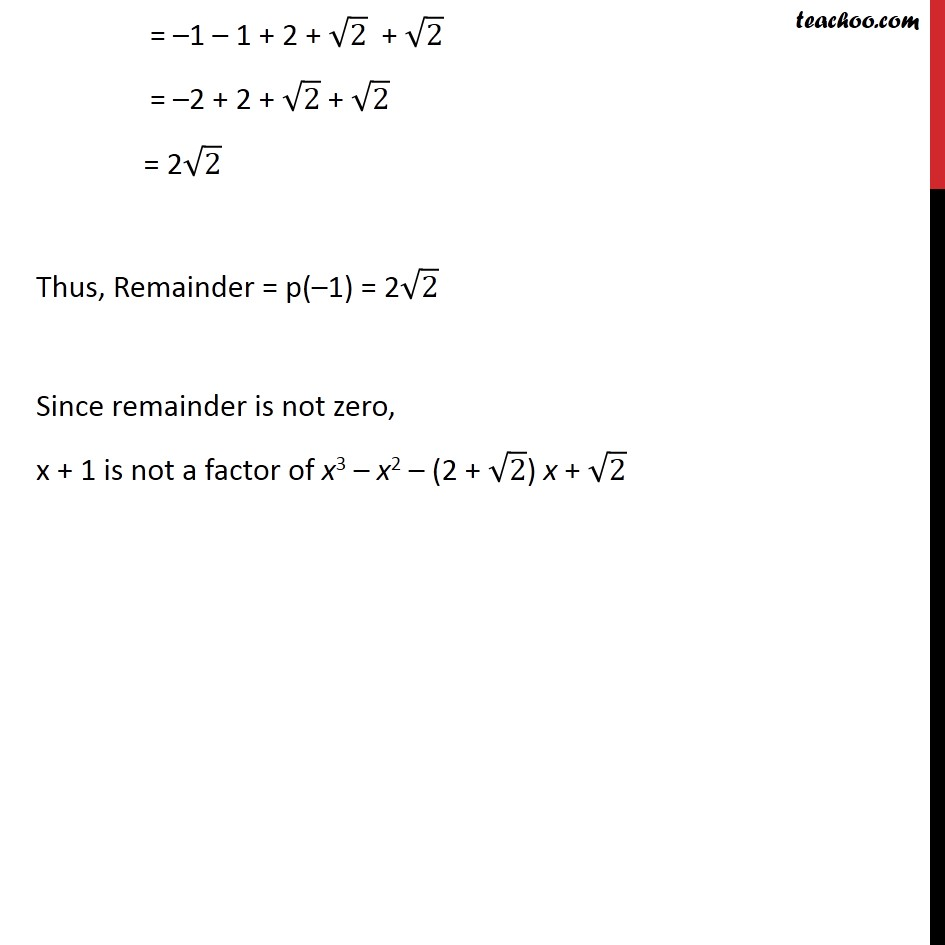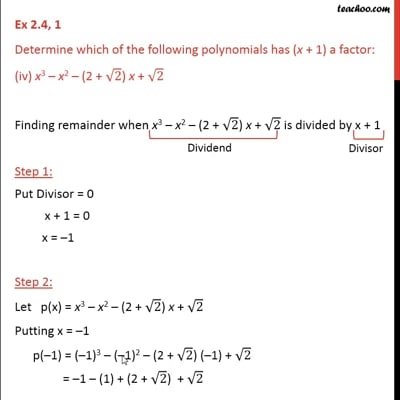Ex 2.4

Chapter 2 Class 9 Polynomials
Serial order wiseThis video is only available for Teachoo black users

Maths Crash Course - Live lectures + all videos + Real time Doubt solving!

### Transcript

Ex 2.4, 1 Determine which of the following polynomials has (x + 1) a factor: (iv) x3 x2 (2 + 2) x + 2 Finding remainder when x3 x2 (2 + 2) x + 2 is divided by x + 1 Step 1: Put Divisor = 0 x + 1 = 0 x = 1 Step 2: Let p(x) = x3 x2 (2 + 2) x + 2 Putting x = 1 p( 1) = ( 1)3 ( 1)2 (2 + 2) ( 1) + 2 = 1 (1) + (2 + 2) + 2 = 1 1 + 2 + 2 + 2 = 2 + 2 + 2 + 2 = 2 2 Thus, Remainder = p( 1) = 2 2 Since remainder is not zero, x + 1 is not a factor of x3 x2 (2 + 2) x + 2